Courses

# RD Sharma Solutions (Part - 1) - Ex-14.1, Lines and Angles, Class 7, Math Class 7 Notes | EduRev

## RD Sharma Solutions for Class 7 Mathematics

Created by: Abhishek Kapoor

## Class 7 : RD Sharma Solutions (Part - 1) - Ex-14.1, Lines and Angles, Class 7, Math Class 7 Notes | EduRev

The document RD Sharma Solutions (Part - 1) - Ex-14.1, Lines and Angles, Class 7, Math Class 7 Notes | EduRev is a part of the Class 7 Course RD Sharma Solutions for Class 7 Mathematics.
All you need of Class 7 at this link: Class 7

#### Question 1:

Write down each pair of adjacent angles shown in Fig.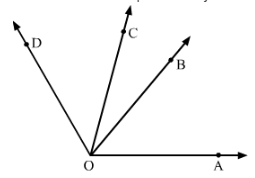Adjacent angles are the angles that have a common vertex and a common arm.
Following are the adjacent angles in the given figure:

∠DOC and ∠BOC
∠COB and ∠BOA

#### Question 2:

In Fig., name all the pairs of adjacent angles.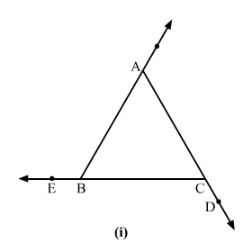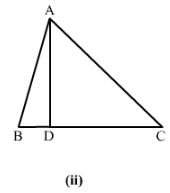In figure (i), the adjacent angles are:

∠EBA and∠ABC
∠ACB and ∠BCF

In figure (ii), the adjacent angles are:

∠∠BDA and ∠∠CDA

#### Question 3:

In figure, write down: (i) each linear pair (ii) each pair of vertically opposite angles.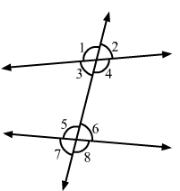(i) Two adjacent angles are said to form a linear pair of angles if their non-common arms are two opposite rays.
∠1 and ∠3
∠1 and ∠2
∠4 and ∠3
∠4 and ∠2
∠5 and ∠6
∠5 and ∠7
∠6 and ∠8
∠7 and ∠8

(ii) Two angles formed by two intersecting lines having no common arms are called vertically opposite angles.
∠1 and ∠4
∠2 and ∠3
∠5 and ∠8
∠6 and ∠7

#### Question 4:

Are the angles 1 and 2 given in Fig. adjacent angles?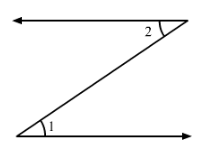No, because they have no common vertex.

#### Question 5:

Find the complement of each of the following angles:
(i) 35°
(ii) 72°
(iii) 45°
(iv) 85°

Two angles are called complementary angles if the sum of those angles is 90°.

Complementary angles of the following angles are:

(i) 90°−35°=55°
(ii) 90°−72°=18°
(iii) 90°−45°=45°
(iv) 90°−85°=5°

#### Question 6:

Find the supplement of each of the following angles:
(i) 70°
(ii) 120°
(iii) 135°
(iv) 90°

Two angles are called supplementary angles if the sum of those angles is 180°.
Supplementary angles of the following angles are:

(i) 180° − 70° = 110°
(ii) 180° − 120° = 60°
(iii) 180° − 135° = 45°
(iv) 180° − 90° = 90°

#### Question 7:

Identify the complementary and supplementary pairs of angles from the following pairs:
(i) 25°, 65°
(ii) 120°, 60°
(iii) 63°, 27°
(iv) 100°, 80°

Since
(i) 25°+65°=90° , therefore this is complementary pair of angle.
(ii) 120°+ 60°= 180°, therefore this is supplementary pair of angle.
(iii) 63°+27°= 90°, therefore this is complementary pair of angle.
(iv) 100°+ 80°= 180° , therefore this is supplementary pair of angle.

Therefore, (i) and (iii) are the pairs of complementary angles and (ii) and (iv) are the pairs of supplementary angles.

#### Question 8:

Can two angles be supplementary, if both of them be
(i) obtuse?
(ii) right?
(iii) acute?

(i) No, two obtuse angles cannot be supplementary.
(ii) Yes, two right angles can be supplementary. (∵∠90°+∠90°=∠180°)
(iii) No, two acute angles cannot be supplementary.

#### Question 9:

Name the four pairs of supplementary angles shown in Fig.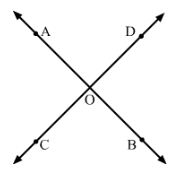Following are the supplementary angles:
∠AOC and ∠COB
∠BOC and ∠DOB
∠BOD and ∠DOA
∠AOC and ∠DOA

#### Question 10:

In Fig., A, B, C are collinear points and ∠DBA = ∠EBA.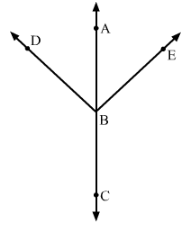(i) Name two linear pairs
(ii) Name two pairs of supplementary angles.

(i) Linear pairs:
∠ABD and ∠DBC
∠ABE and ∠EBC

Because every linear pair forms supplementary angles, these angles are:
∠ABD and ∠DBC
∠ABE and ∠EBC

#### Question 11:

If two supplementary angles have equal measure, what is the measure of each angle?

Let and y be two supplementary angles that are equal.
∠x=∠y
According to the question,
∠x+∠y=180°
⇒∠x+∠x=180°
⇒2∠x=180°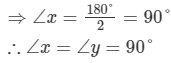#### Question 12:

If the complement of an angle is 28°, then find the supplement of the angle.

Let x be the complement of the given angle 28°28°.
∴ ∠x+28°=90°
⇒∠x=90°−28°=62°

So, supplement of the angle = 180°−62°=118°180°-62°=118°

#### Question 13:

In Fig. 19, name each linear pair and each pair of vertically opposite angles: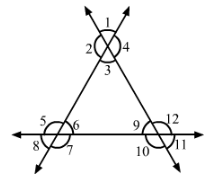Two adjacent angles are said to form a linear pair of angles if their non-common arms are two opposite rays.

∠1 and ∠2
∠2 and ∠3
∠3 and ∠4
∠1 and ∠4
∠5 and ∠6
∠6 and ∠7
∠7 and ∠8
∠8 and ∠5
∠9 and ∠10
∠10 and ∠11
∠11 and ∠12
∠12 and ∠9

Two angles formed by two intersecting lines having no common arms are called vertically opposite angles.
∠1 and ∠3
∠4 and ∠2
∠5 and ∠7
∠6 and ∠8
∠9 and ∠11
∠10 and ∠12

#### Question 14:

In Fig., OE is the bisector of ∠BOD. If ∠1 = 70°, find the magnitudes of ∠2, ∠3 and ∠4.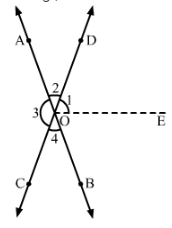Since OE is the bisector of BOD,
DOE=EOB

2+1+EOB=180°                 (Linear Pair)
2+21=180°              (1=EOB)
2=180°21=180°2×70°=180°140°=40°
4=2=40°                (Vertically opposite angles)
3=DOB=1+EOB=70°+70°=140°            [3=DOB (Vertically opposite angles)

97 docs

,

,

,

,

,

,

,

,

,

,

,

,

,

,

,

,

,

,

,

,

,

,

,

,

,

,

,

,

,

,

;